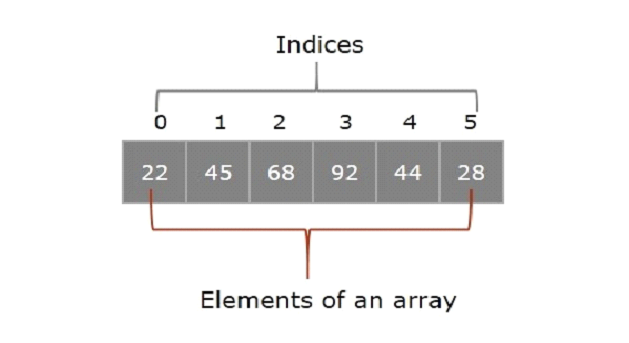# Write a program to find the index of particular element in an array in javascript?

Array is an object which contains multiple values of the same datatype in a sequential order. In other words, we can say that an array is a special type of object in the JavaScript.

### Syntax

We can create an array in two ways in JavaScript. The syntax are given below −

var arr = [val1, val2, …];
var arr = new Array(“val1”, “val2”, …)


Now let us look at a simple JavaScript program to create an array and print its index values −

var arr = [1,2,3,4,5];
document.write(arr);


Here, the program returns the content in 0th index of an array, i.e. ‘1’, as the output.

## Elements of an Array

Elements are the values present in an array and the index indicates the position of the element in the array.

Each entity (value) of an array is known as an element and the position of these elements is indicated by a numerical value known as the index. The index starts with 0 and ends with the length of the array-1. In the following diagram, 22 is an element of an array, and its index is 0.## Using for loop

Using a for loop and an if condition, we can find the index of an element in the given array.

The for loop is used to traverse through all the elements of the given array, where ‘i’ represents the indices of the array and using the conditional statement if we can match each element with the desired one (element to be searched) and print the index of the matched element.

For finding the index of a particular element in an array we have various types of ways. Let’s discuss them one by one with suitable examples.

### Example 1

In the following example, we are taking hard coded input value and finding the index of that value using a for loop. We first create an array with some elements, then use a for loop to fetch all the array elements and we make use of conditional statements in order to compare the elements.

<!DOCTYPE html>
<html lang="en">
<title>Array Index</title>
<body>
<script>
var subjects = ["English", "Hindi", "Science", "Mathematics"];
for(let i = 0; i<subjects.length; i++){

if(subjects[i] == 'Hindi')
document.write("Index of particular element is = " + i);
}
</script>
</body>
</html>


### Example 2

Let’s look at another example, in which we will accept the input from user and iteratively compare the elements to find the index of the inputted element −

<!DOCTYPE html>
<html lang="en">
<title>Array Index</title>
<body>
<script>
var subjects = ["English", "Hindi", "Science", "Mathematics"];
var sname = prompt("Enter a subject name: ")
for(let i = 0; i<subjects.length; i++){
if(subjects[i] == sname)
document.write("Index of particular element is = " + i);
}
</script>
</body>
</html>


## Using the indexOf() method

The indexOf() method in JavaScript is used to retrieve the index of a particular element of an array.

The JavaScript indexOf() method accepts a string value representing the element we need to find, as a parameter and returns the position of that particular element. If the value is not found, then it’s returns -1. Following is the syntax of this method −

### Syntax

arr.indexOf(char)


### Example

In the following program, we use a built-in method in JavaScript called indexOf() which finds the index of the elements in an array.

<!DOCTYPE html>
<html lang="en">
<title>Array Index</title>
<body>
<script>
var color = ['red', "black", 'green', 'orange', 'yellow'];
var cname = prompt("Enter a color name: ");

var index = color.indexOf(cname);
document.write("Index of particular element is = " + index);
</script>
</body>
</html>


## Using the findIndex() method

findindex() is a built-in method in JavaScript, we can find the index of the particular elements an array using this method.

### Syntax

Following is the syntax of this method −

var index = arr.findIndex(varibale => variable.property(=,>,===) value;


### Example

In the following example, we find the index of a specified element using findIndex() method. Using this method, we locate the index of the inputted element, that we take from the users on the terminal, and print the output.

<!DOCTYPE html>
<html lang="en">
<title>Array Index</title>
<body>
<script>
var arr = [1,2,3,4,5,6];
var num = Number(prompt("Enter a number:-"));
var index = (element) => element === num;
console.log(arr.findIndex(index));
document.write("Index of particular element is = "+ arr.findIndex(index));
</script>
</body>
</html>


Updated on: 29-Aug-2022

762 Views